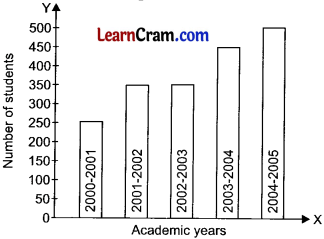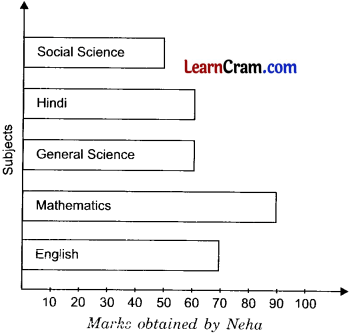# DAV Class 6 Maths Chapter 16 Worksheet 3 Solutions

The DAV Class 6 Maths Book Solutions and DAV Class 6 Maths Chapter 16 Worksheet 3 Solutions of Statistics offer comprehensive answers to textbook questions.

## DAV Class 6 Maths Ch 16 WS 3 Solutions

Question 1.Bar graph of the number of students in
class VI of a school during academic year
2000-2001 to 2004-2005.
(a) How many students were there in the year 2001-2002?
350 students were there in the year 2001-2002.

(b) How many students were there in the year 2003-2004?
450 students were there in the year 2003-2004.

(c) Name the years in which number of students were the same.
In 2001-2002 and 2002-2003, the number of students were same.

(d) In which year, the number of students was maximum? Also how many were there in this year?
In the year 2004-2005, the number of students were maximum. There were 500 students in this year.Question 2.
Observe the bar graph showing the marks obtained by Neha in different subjects in her annual examinations.(a) What information is given by the bar graph?
The above bar graph shows the marks obtained by Neha in the annual examination.

(b) In which subject did Neha score maximum marks?
Neha obtained maximum marks in Mathematics.

(c) How many marks did Neha score in:
(i) Hindi
(ii) Mathematics?